Since we launched the Easel Apps API for early access last year, we’ve received a lot of excellent feedback from developers on how it could be improved. One of the apps that makes a good case study for improving the API is Vojtěch Kadlec’s puzzle generator. As I was considering how we could better support an app like his, I became a little curious about how one would go about generating puzzle pieces with unique shapes. I couldn’t resist tackling the problem, and I thought I’d share my approach to solving it.

Let’s start by playing with the final product. Type numbers or click the arrows in the text boxes to generate new puzzles.

Standard jigsaw puzzles are essentially m x n matrices, with m rows and n columns. The key characteristic of these grids is that each interior edge (where the jigsaw cuts) is a curve with a single “knob:”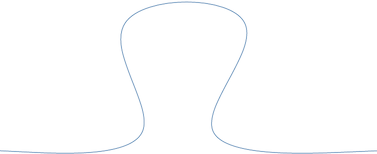If we can generate a curve with a knob, then we can generate a puzzle by placing one such curve on every interior edge. If we can also randomize the shape of the curve a bit each time, then we can generate a random puzzle. So how can we generate a random curve?

Let’s experiment. Try dragging the blue circles around in the box below until the curve resembles the one shown above for the edge of a puzzle piece. The circles will turn green when they enter a good region.

Try me!

Nice job. The puzzle edge that you created is a B-spline, with control point duplication on the ends. (We’re using the fantastic D3 library to generate the curve from the points you dragged around.) You might have noticed that each point can move around a pretty good amount in its local region and still produce a nice-looking puzzle edge. This lays the basis for how we can generate random edges: slightly vary the locations for each control point within well-defined regions.

Here’s the function we’ll use to generate the control points for a single edge. In order to make each edge’s curve easier to position, we express all points as percentage offsets from the edge’s corner. The first coordinate of each point represents its percentage displacement in the direction of the edge. The second coordinate represents its percentage displacement perpendicular to the edge. In order to make some of the knobs point inward and some of them point outward, we also randomly invert each of the second coordinates in about half of the edges.

var edgeDistributions = (function() {
var randomBetween = function(min, max) {
return Math.random() * (max - min) + min;
};

// These are good regions in which to displace control points
// at the bottom of the knob
var baselineOffsets = {
xMin: 51,
xMax: 62,
yMin: -15,
yMax: 5
};

// These are good regions in which to displace control points
// at the top of the knob
var upperOffsets = {
xMin: 20,
xMax: 30,
yMin: 20,
yMax: 44
};

return function() {
var point1 = [0, 0];
var point2 = [
randomBetween(baselineOffsets.xMin, baselineOffsets.xMax),
randomBetween(baselineOffsets.yMin, baselineOffsets.yMax)
];
var point3 = [
randomBetween(upperOffsets.xMin, upperOffsets.xMax),
randomBetween(upperOffsets.yMin, upperOffsets.yMax)
];
var point4 = [
randomBetween(100 - upperOffsets.xMax, 100 - upperOffsets.xMin),
randomBetween(upperOffsets.yMin, upperOffsets.yMax)
];
var point5 = [
randomBetween(100 - baselineOffsets.xMax, 100 - baselineOffsets.xMin),
randomBetween(baselineOffsets.yMin, baselineOffsets.yMax)
];
var point6 = [100, 0];

var points = [point1, point2, point3, point4, point5, point6];

// Choose whether to point inward or outward
var sign = Math.random() < 0.5 ? -1 : 1;

return points.map(function(p) {
return [p / 100, p * sign / 100];
});
}
})();


Invoking this function just gives us the points to generate one lonely edge: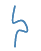We can’t do much with one edge, so we need to generate a lot more of them. For an m x n puzzle, we need n(m - 1) knobby edges going across to form the row boundaries, and m(n - 1) knobby edges going vertically to form the column boundaries. We also need 2m flat edges on the sides and 2n flat edges for the top and bottom. Since we expressed our edge distributions as offsets, we can use a single function to generate both the horizontal and vertical edges and worry about orienting them later:

// Builds an (m + 1) x n matrix of edge shapes. The first and last rows
// are straight edges.
var buildDistributions = function(m, n) {
var lineGroups = [];
var lines = [];
var points, i, j;

for (j = 0; j < n; j++) {
lines.push([[0, 0], [1,0]]);
}
lineGroups.push(lines);

for (i = 1; i < m; i++) {
lines = [];
for (j = 0; j < n; j++) {
lines.push(edgeDistributions());
}
lineGroups.push(lines);
}

lines = [];
for (j = 0; j < n; j++) {
lines.push([[0, 0], [1,0]]);
}
lineGroups.push(lines);

return lineGroups;
};


We can invoke this function once to generate an array of horizontal row edges:

  var buildRows = function(rowCount, columnCount, rowHeight, columnWidth) {
var rows = buildDistributions(rowCount, columnCount);

offsetPoints(rows, function(point, j, i) {
return offsetPoint(point, j, i, columnWidth, rowHeight);
});

return rows;
}


# ↓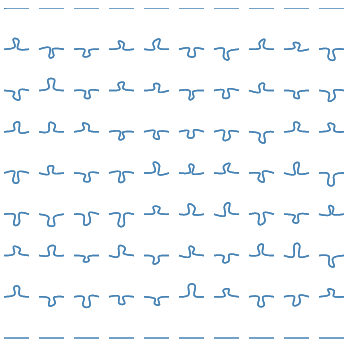And we can invoke it again, inverting the row/column parameters and transposing the points to build an array of vertical column edges:

  var buildColumns = function(rowCount, columnCount, rowHeight, columnWidth) {
var columns = buildDistributions(columnCount, rowCount);

offsetPoints(columns, function(point, j, i) {
return offsetPoint(transposePoint(point), i, j, columnWidth, rowHeight);
});

return columns;
};


# ↓Now that we have all of our edges, we need to assemble them in the proper arrangement. There’s one hidden wrinkle of complexity here: since we’re making a puzzle to be physically cut out of material, we need to build separate paths for each piece so that the pieces can be spaced far enough apart that the cutting tool following the edges won’t accidentally remove material from adjacent pieces.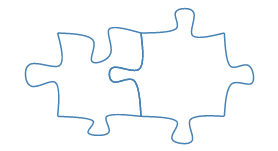Each interior knobby edge of a puzzle is shared by two puzzle pieces. We can use our rows and columns arrays to refer to specific edges and re-use them when needed as we move through the logical rows and columns of our puzzle:

  var buildPieces = function(rowCount, columnCount) {
var rowHeight = height / rowCount;
var columnWidth = width / columnCount;
var pieces = [];

var rows = buildRows(rowCount, columnCount, rowHeight, columnWidth);
var columns = buildColumns(rowCount, columnCount, rowHeight, columnWidth);

for (var rowIndex = 1; rowIndex <= rowCount; rowIndex++) {
for (var columnIndex = 0; columnIndex < columnCount; columnIndex++) {
var edges = [];
edges.push(rows[rowIndex - 1][columnIndex]);
edges.push(columns[columnIndex + 1][rowIndex - 1]);
edges.push(rows[rowIndex][columnIndex].slice().reverse());
edges.push(columns[columnIndex][rowIndex - 1].slice().reverse());

pieces.push(edges);
}
}

return pieces;
};


Now we have points for all four edges of every piece in our puzzle, offset to their correct locations. From here we’ll just leverage D3’s line helper to convert our point arrays to SVG path data strings, wrap everything in the proper tags, and–tada!–a random puzzle!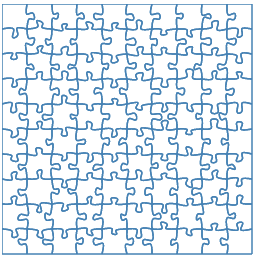You can view the full source, including the offset functions, and play with the generator in the bl.ock.

In a future post, I’ll show how we can build on this to make puzzles out of arbitrary designs in Easel.